Get instant live expert help with Excel or Google Sheets“My Excelchat expert helped me in less than 20 minutes, saving me what would have been 5 hours of work!”

#### Post your problem and you'll get expert help in seconds

Your message must be at least 40 characters
Our professional experts are available now. Your privacy is guaranteed.

# Student class enrollment with table

While working with Excel, we are able to organize our data to suit our preference.  This tutorial will assist all levels of Excel users in tracking student class enrollment with a table using the IF and COUNTIF functions.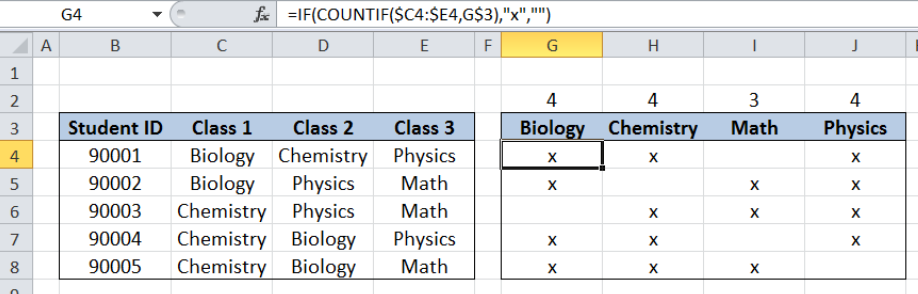Figure 1. Final result: Student class enrollment with table

Final formula: `=IF(COUNTIF(\$C4:\$E4,G\$3),"x","")`

## Syntax of IF Function

IF function evaluates a given logical test and returns a TRUE or a FALSE

`=IF(logical_test, [value_if_true], [value_if_false])`

• logical_test any value that we want to evaluate whether TRUE or FALSE
• The arguments “value_if_true” and “value_if_false” are optional.  If left blank, the function will return TRUE if the logical test is met, and FALSE if otherwise.

## Syntax of COUNTIF Function

COUNTIF returns the count or number of values in a specified range based on a given condition

`=COUNTIF(range,criteria)`

• range – the data range that will be evaluated using the criteria
• criteria – the criteria or condition that determines which cells will be counted

## Setting up Our Data

Here we have data on the enrolled classes per student.  Our table consists of Student ID (column B), Class 1 (column C), Class 2 (column D) and Class 3 (column E).  We want to organize the enrollment data to easily show the students who enrolled in each class, and the total count of enrollees per class.

We allocate one column per class in columns G to J: Biology (column G), Chemistry (column H), Math (column I) and Physics (column J).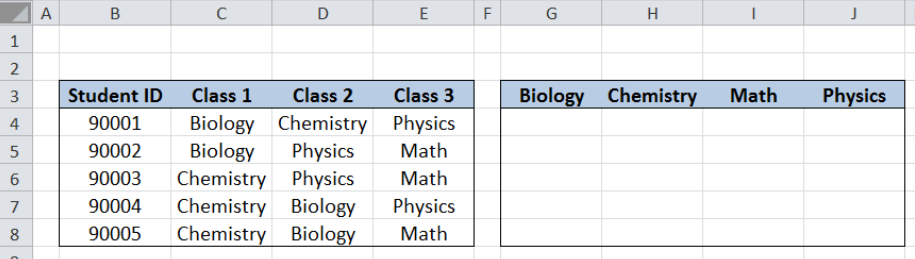Figure 2. Sample data for student class enrollment with table

## Tracking of student class enrollment

We want to mark the specific classes for each student in our list.  We can do this by using the IF and COUNTIF functions. Let us follow these steps:

Step 1.  Select cell G4

Step 2.  Enter the formula: `=IF(COUNTIF(\$C4:\$E4,G\$3),"x","")`

Step 3:  Press ENTER

Step 4:  Copy the formula in cell G4 to cells G4:J8 by clicking the “+” icon at the bottom-right corner of cell G4 and dragging it down to G8, then right towards J8.

The dollar signs “\$” in the formula fix the cells so that we can easily copy and paste the formula to other cells.

Our formula tests one condition.  It returns an “x” when the value is TRUE, and blank if otherwise.

The logical test is determined by the COUNTIF function: COUNTIF(\$C4:\$E4,G\$3).  COUNTIF counts the cells in the range C4:E4 which has a value equal to G3 Biology”.  Since Biology is found in cell C4, COUNTIF returns the value “1”.

As a result, our logical test returns TRUE and the IF function returns “x”.  Cell G4 is marked with an “x”, which means that Student 90001 has enrolled in the Biology class.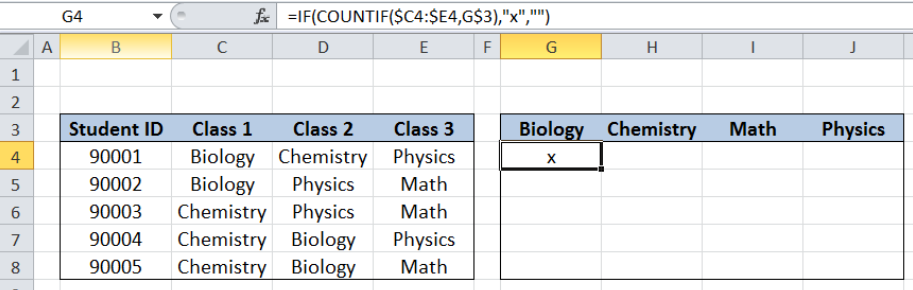Figure 3. Entering the formula using IF and COUNTIF

Copying the formula to the succeeding cells marks columns G to J with an “x” for enrolled classes.  This way, the enrollment data is presented in a clearer and simpler manner.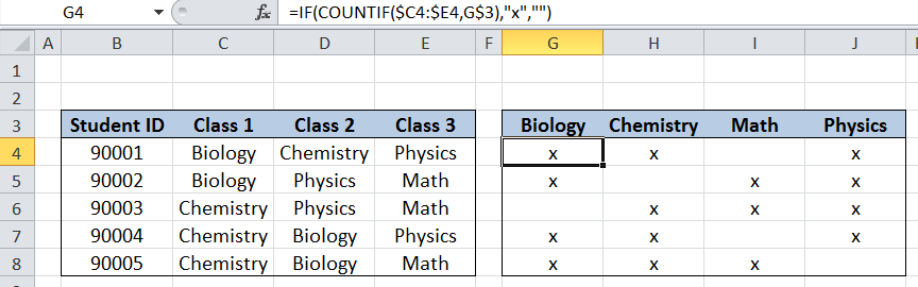Figure 4. Output: Student class enrollment with table

## Determine enrollment count

We want to count the enrollment per class and record it in cells G2:J2, just above the headers for the classes.  Let us follow these steps:

Step 1.  Select cell G2

Step 2.  Enter the formula: `=COUNTIF(G4:G8,"x")`

Step 3:  Press ENTER

Step 4:  Copy the formula in cell G2 to cells H2:J2 by clicking the “+” icon at the bottom-right corner of cell G2 and dragging to the right towards J2.

Our formula counts the cells marked with an “x” per column or per class.  Below table shows the count of enrollees per class.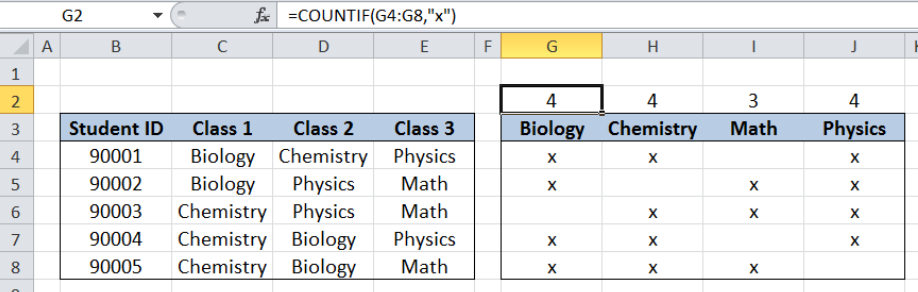Figure 5. Output: Class enrollment count using COUNTIF

Most of the time, the problem you will need to solve will be more complex than a simple application of a formula or function. If you want to save hours of research and frustration, try our live Excelchat service! Our Excel Experts are available 24/7 to answer any Excel question you may have. We guarantee a connection within 30 seconds and a customized solution within 20 minutes.

### Did this post not answer your question? Get a solution from connecting with the expert.Another blog reader asked this question today on Excelchat:## Subscribe to Excelchat.coAnother blog reader asked this question today on Excelchat: